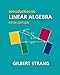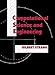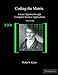# MATH09003 2017 Applied Linear Algebra

### General Details

Full Title
Applied Linear Algebra
Transcript Title
Applied Linear Algebra
Code
MATH09003
Attendance
N/A %
Subject Area
MATH - Mathematics
Department
MENG - Mech. and Electronic Eng.
Level
09 - NFQ Level 9
Credit
05 - 05 Credits
Duration
Semester
Fee
Start Term
2017 - Full Academic Year 2017-18
End Term
9999 - The End of Time
Author(s)
Leo Creedon, Donny Hurley
Programme Membership
SG_ESENS_E09 202000 Certificate in Sensors for Autonomous Vehicles SG_ECONN_O09 202000 Postgraduate Diploma in Engineering in Connected and Autonomous Vehicles SG_ECONN_M09 202000 Master of Engineering in Connected and Autonomous Vehicles SG_KDATA_M09 202100 Master of Science in Data Science SG_ECONN_M09 202100 Master of Engineering in Connected and Autonomous Vehicles SG_ECONN_O09 202100 Postgraduate Diploma in Engineering in Connected and Autonomous Vehicles SG_ECOFT_O09 202100 Postgraduate Diploma in Engineering in Connected and Autonomous Vehicles SG_EAUTO_E09 202100 Certificate in Automotive Artificial Intelligence
Description

The subject covers the linear algebra required for post-graduate engineering courses.  The learner will gain the expertise to interpret the linear algebra models used in the engineering literature. It will also enable learners to model problems using linear algebra methods.

### Learning Outcomes

On completion of this module the learner will/should be able to;

1.

Solve systems of linear equations and analyse the solutions.

2.

Analyse affine transformations in three dimensions.

3.

Interpret the linear algebra in state of the art research publications and reproduce findings.

4.

Explain the use of vector spaces in analysing solutions to systems of equations.

5.

Use projections to find the least squares solution of overdetermined systems.

6.

Decompose matrices into their singular value decompositions and interpret.

7.

Apply matrices to the Fourier transform, graphs and networks.

### Teaching and Learning Strategies

A lecture will be provided each week. In advance of the lecture, the learner will be asked to review material that is relevant to the lecture so that they get the maximum learning from that lecture.

The project work will challenge the learner to master concepts beyond those covered in the theory lecture.

### Module Assessment Strategies

A terminal exam and continuous assessment will be used to assess the module.

To reinforce the theoretical principles covered in lectures, learners will participate in project work.

The learner will complete a final exam at the end of the semester.

The learner is required to pass both the projects and terminal examination element of this module.

### Repeat Assessments

Repeat Exams will be set for Autumn of each year.

Repeat project work can be submitted at the repeat exam sitting.

### Indicative Syllabus

Review solutions of linear equations using Gauss Elimination / Back-substitution / Elementary Row operations / pivots / REF / Gauss-Jordan elimination / RREF

- Consistency and systems with unique/multiple/zero solutions

- (Geometry of Solutions) (solutions of equations as points, lines and planes)

- Complete solution to AX = B (column space containing b, rank and nullspace of A and special solutions to AX = 0 from row reduced R)

Matrix Algebra

Review addition and multiplication of matrices and vectors and their transposes.

- Inverse matrices. Calculation via Gauss Elimination. Invertibility.

- Block multiplication

3D Vectors/Rotation

- Inner product, length, orthogonality cosine rule/angles, cross product

- Rotation matrices, Euler angles and non-commutativity

- Projection, reflection, scaling

- Lines / planes in 3 dimensions

- Linear transformations and change of basis (dual basis)

Vector spaces and subspaces

- Basis and dimension / linear independence and span

- Four fundamental subspaces ( row, column, right-left nullspaces, and their bases)

Least squares solutions, overdetermined systems (closest line by understanding projections)

Orthogonalization by Gram-Schmidt (factorization into A = QR)

Orthonormal matrices  (rotation matrices as)

Determinants / as volume / cofactor formula / applications to inverses / calculation via Gauss elimination

Eigenvalues / SVD

- Eigenvalues and eigenvectors (diagonalizing and computing powers of matrices)

- Matrix exponentials. Solving difference and differential equations

- Symmetric matrices and positive definite matrices (real eigenvalues and orthogonal eigenvectors, tests for x'Ax > 0, applications) A=QLQT

- Singular Value Decomposition (SVD)

- The Moore-Penrose Pseudoinverse. Least solution underdetermined system.

LU Factorisation

Skew-symmetric matrices / skew matrices

Fourier matrices / fast Fourier transform

Graphs and networks

Kronecker product

### Coursework & Assessment Breakdown

Coursework & Continuous Assessment
60 %
End of Semester / Year Formal Exam
40 %

### Coursework Assessment

Title Type Form Percent Week Learning Outcomes Assessed
1 CA 1 Continuous Assessment Assessment 30 % Week 6 1,2,4
2 Project Continuous Assessment Project 30 % Week 12 1,2,3,4,5,6,7

### End of Semester / Year Assessment

Title Type Form Percent Week Learning Outcomes Assessed
1 Terminal Exam Final Exam Closed Book Exam 40 % End of Semester 1,2,3,4,5,6,7

Type Location Description Hours Frequency Avg Workload
Lecture Lecture Theatre Lecture 2 Weekly 2.00
Laboratory Practical Computer Laboratory Laboratory Practical 2 Fortnightly 1.00
Independent Learning Not Specified Independent Learning 7 Weekly 7.00
Total Full Time Average Weekly Learner Contact Time 3.00 Hours

Type Location Description Hours Frequency Avg Workload
Lecture Online Theory Lecture 1 Weekly 1.00
Independent Learning Not Specified Independent Learning 8.5 Weekly 8.50
Laboratory Practical Online Online lab 0.5 Weekly 0.50
Total Online Learning Average Weekly Learner Contact Time 1.50 Hours

### Required & Recommended Book List2016-08-11 Introduction to Linear Algebra Wellesley-Cambridge Press
ISBN 0980232775 ISBN-13 97809802327762007-11-01 Computational Science and Engineering Wellesley-Cambridge Press
ISBN 0961408812 ISBN-13 9780961408817

Computational Science and Engineering This book encompasses the full range of computational science and engineering from modelling to solution, whether analytic or numerical. Full description2013-09-03 Coding the Matrix: Linear Algebra through Applications to Computer Science Newtonian Press
ISBN 0615880991 ISBN-13 9780615880990

### Module Resources

Journal Resources

x

URL Resources

x

Other Resources

x